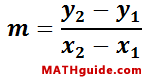Parallel, Perpendicular, or Neither
View the Lesson | MATHguide homepage Updated March 24th, 2018

Status: Waiting for your answers.

Given:Points A(0, 1), B(1, 6), C(5, -11), D(6, -6)Problem:Calculate the slopes of line AB and line CD to determine if they are parallel, perpendicular, or neither.
 mAB =mCD =Characteristic: